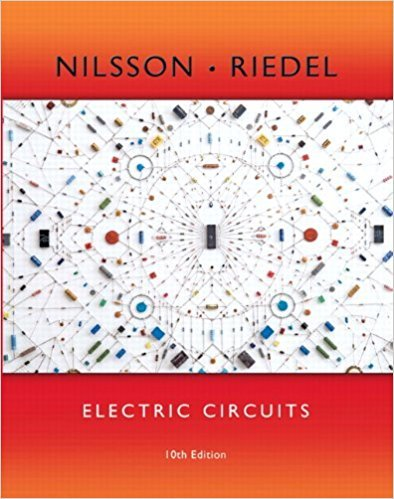×
×

# Solutions for Chapter 12: Introduction to the Laplace Transform## Full solutions for Electric Circuits | 10th Edition

ISBN: 9780133760033Solutions for Chapter 12: Introduction to the Laplace Transform

Solutions for Chapter 12
4 5 0 376 Reviews
23
1
##### ISBN: 9780133760033

This expansive textbook survival guide covers the following chapters and their solutions. This textbook survival guide was created for the textbook: Electric Circuits, edition: 10. Since 56 problems in chapter 12: Introduction to the Laplace Transform have been answered, more than 14692 students have viewed full step-by-step solutions from this chapter. Chapter 12: Introduction to the Laplace Transform includes 56 full step-by-step solutions. Electric Circuits was written by and is associated to the ISBN: 9780133760033.

Key Engineering and Tech Terms and definitions covered in this textbook
×